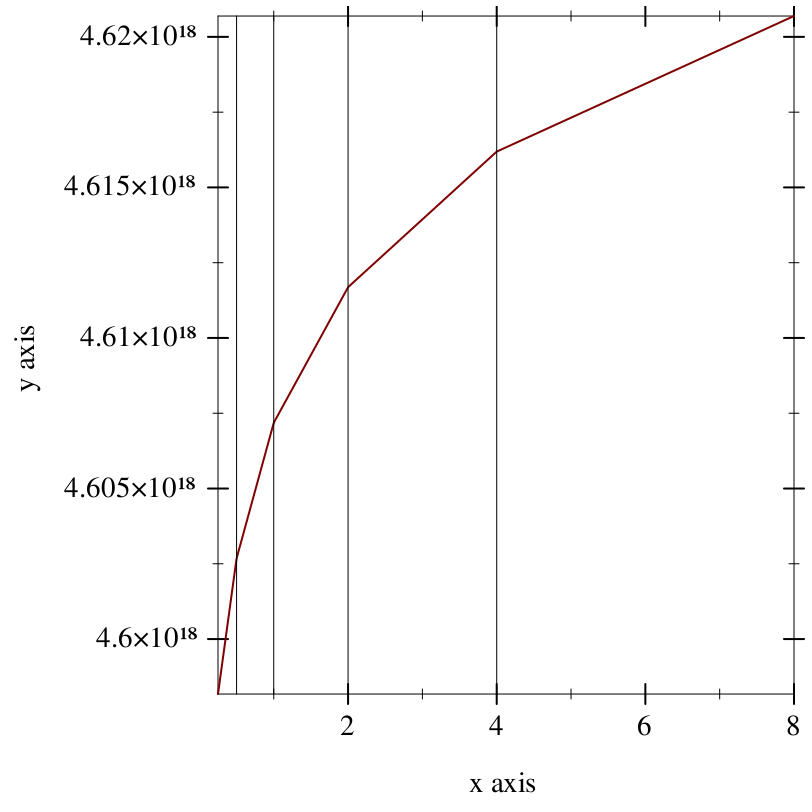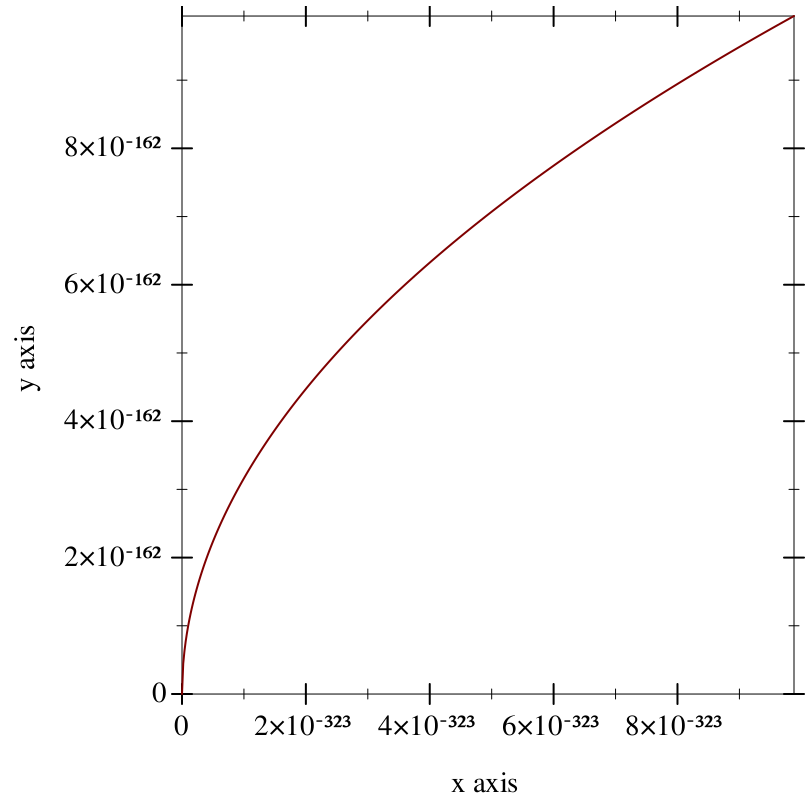8.3

## Unstable Flonums: May Change Without Warning

This library is unstable; compatibility will not be maintained. See Unstable: May Change Without Warning for more information.

 (require unstable/flonum) package: unstable-flonum-lib

You should almost certainly use math/flonum instead of this module, which is more complete and can be used in Typed Racket code.

 procedure(flonum->bit-field x) → (integer-in 0 (- (expt 2 64) 1)) x : flonum?
Returns the bits comprising x as an integer. A convenient shortcut for composing integer-bytes->integer with real->floating-point-bytes.

Examples:
 > (number->string (flonum->bit-field -inf.0) 16) "fff0000000000000" > (number->string (flonum->bit-field +inf.0) 16) "7ff0000000000000" > (number->string (flonum->bit-field -0.0) 16) "8000000000000000" > (number->string (flonum->bit-field 0.0) 16) "0" > (number->string (flonum->bit-field -1.0) 16) "bff0000000000000" > (number->string (flonum->bit-field 1.0) 16) "3ff0000000000000" > (number->string (flonum->bit-field +nan.0) 16) "7ff8000000000000"

 procedure i : (integer-in 0 (- (expt 2 64) 1))
The inverse of flonum->bit-field.

 procedure → (integer-in (- (- (expt 2 63) 1)) (- (expt 2 63) 1)) x : flonum?
Returns the signed ordinal index of x in a total order over flonums.

When inputs are not +nan.0, this function is monotone and symmetric; i.e. if (fl<= x y) then (<= (flonum->ordinal x) (flonum->ordinal y)), and (= (flonum->ordinal (- x)) (- (flonum->ordinal x))).

Examples:
 > (flonum->ordinal -inf.0) -9218868437227405312 > (flonum->ordinal +inf.0) 9218868437227405312 > (flonum->ordinal -0.0) 0 > (flonum->ordinal 0.0) 0 > (flonum->ordinal -1.0) -4607182418800017408 > (flonum->ordinal 1.0) 4607182418800017408 > (flonum->ordinal +nan.0) 9221120237041090560

These properties mean that flonum->ordinal does not distinguish -0.0 and 0.0.

The following plot demonstrates how the density of floating-point numbers decreases with magnitude:

Example:
 > (parameterize ([y-axis-ticks? #f]) (plot (list (function (compose flonum->ordinal exact->inexact) 1/4 8) (y-axis 1/2) (y-axis 1) (y-axis 2) (y-axis 4))))procedure i : (integer-in (- (- (expt 2 63) 1)) (- (expt 2 63) 1))
The inverse of flonum->ordinal.

 procedure x : flonum? y : flonum?
Returns the number of flonums between x and y, excluding one endpoint. Equivalent to (- (flonum->ordinal y) (flonum->ordinal x)).

Examples:
 > (flonums-between 0.0 1.0) 4607182418800017408 > (flonums-between 1.0 2.0) 4503599627370496 > (flonums-between 2.0 3.0) 2251799813685248 > (flonums-between 1.0 +inf.0) 4611686018427387904

 procedure(flstep x n) → flonum? x : flonum? n : exact-integer?
Returns the flonum n flonums away from x, according to flonum->ordinal. If x is +nan.0, returns +nan.0.

Examples:
 > (flstep 0.0 1) 5e-324 > (flstep (flstep 0.0 1) -1) 0.0 > (flstep 0.0 -1) -5e-324 > (flstep +inf.0 1) +inf.0 > (flstep +inf.0 -1) 1.7976931348623157e+308 > (flstep -inf.0 -1) -inf.0 > (flstep -inf.0 1) -1.7976931348623157e+308 > (flstep +nan.0 1000) +nan.0

 procedure(flnext x) → flonum? x : flonum?
Equivalent to (flstep x 1).

 procedure(flprev x) → flonum? x : flonum?
Equivalent to (flstep x -1).

 value
 value
 value
 value
The rational flonums with maximum and minimum magnitude.

Examples:
 > (list -max.0 +max.0 -min.0 +min.0) '(-1.7976931348623157e+308 1.7976931348623157e+308 -5e-324 5e-324) > (plot (function sqrt 0 (* 20 +min.0)))